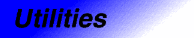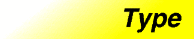pairCategory: utilities Component type: type

Description

Pair<T1,T2> is a heterogeneous pair: it holds one object of type T1 and one of type T2. A pair is much like a Container, in that it "owns" its elements. It is not actually a model of Container, though, because it does not support the standard methods (such as Iterators) for accessing the elements of a Container.

Functions that need to return two values often return a pair.

Example

pair<bool, double> result = do_a_calculation();
if (result.first)
do_something_more(result.second);
else
report_error();

Definition

Defined in the standard header utility, and in the nonstandard backward-compatibility header pair.h.

Template parameters

Parameter Description Default
T1 The type of the first element stored in the pair
T2 The type of the second element stored in the pair

Type requirements

T1 and T2 must both be models of Assignable. Additional operations have additional requirements. Pair's default constructor may only be used if both T1 and T2 are DefaultConstructible, operator== may only be used if both T1 and T2 are EqualityComparable, and operator< may only be used if both T1 and T2 are LessThanComparable.

None.

Members

Member Where defined Description
first_type pair See below.
second_type pair See below.
pair() pair The default constructor. See below.
pair(const first_type&, const second_type&) pair The pair constructor. See below.
pair(const pair&) Assignable The copy constructor
pair& operator=(const pair&) Assignable The assignment operator
first pair See below.
second pair See below.
bool operator==(const pair&, const pair&) pair See below.
bool operator<(const pair&, const pair&) pair See below.
template <class T1, class T2>
pair<T1, T2> make_pair(const T1&, const T2&)
pair See below.

New members

These members are not defined in the Assignable requirements, but are specific to pair.

Member Description
first_type The type of the pair's first component. This is a typedef for the template parameter T1
second_type The type of the pair's second component. This is a typedef for the template parameter T2
pair() The default constructor. It uses constructs objects of types T1 and T2 using their default constructors. This constructor may only be used if both T1 and T2 are DefaultConstructible.
pair(const first_type& x, const second_type& y) The pair constructor. Constructs a pair such that first is constructed from x and second is constructed from y.
first Public member variable of type first_type: the first object stored in the pair.
second Public member variable of type second_type: The second object stored in the pair.
template <class T1, class T2>
bool operator==(const pair<T1,T2>& x,
const pair<T1,T2>& y);
The equality operator. The return value is true if and only the first elements of x and y are equal, and the second elements of x and y are equal. This operator may only be used if both T1 and T2 are EqualityComparable. This is a global function, not a member function.
template <class T1, class T2>
bool operator<(const pair<T1,T2>& x,
const pair<T1,T2>& y);
The comparison operator. It uses lexicographic comparison: the return value is true if the first element of x is less than the first element of y, and false if the first element of y is less than the first element of x. If neither of these is the case, then operator< returns the result of comparing the second elements of x and y. This operator may only be used if both T1 and T2 are LessThanComparable. This is a global function, not a member function.
template <class T1, class T2>
pair<T1, T2> make_pair(const T1& x, const T2& x)
Equivalent to pair<T1, T2>(x, y). This is a global function, not a member function. It exists only for the sake of convenience.